# Coordinate Systems and Axes Conventions

## Local Coordinate System

Local coordinate system is a right-handed cartesian coordinate system of a single reconstruction. It’s based on camera coordinate system. The local camera coordinate system of an image is defined in a way that the X axis points to the right, the Y axis to the bottom, and the Z axis to the front as seen from the image.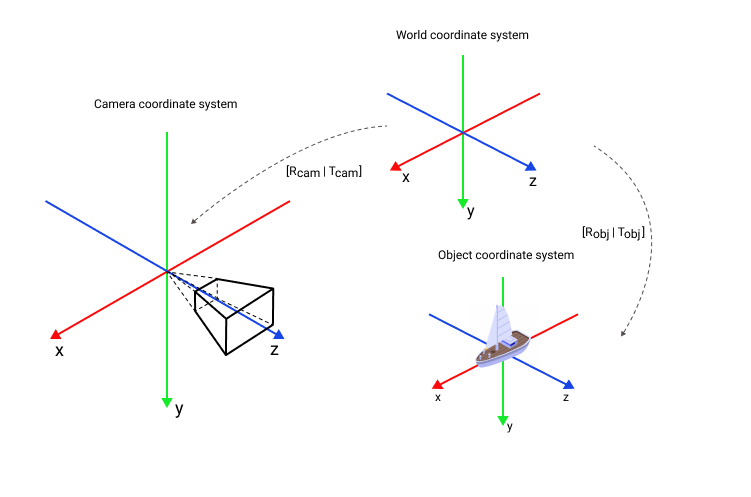The local reconstruction coordinate system has no metric scale. Each reconstruction has a unique coordinate system with its own scale.

## Geographic Coordinate System

AC supports two geographic coordinate systems ECEF and ENU.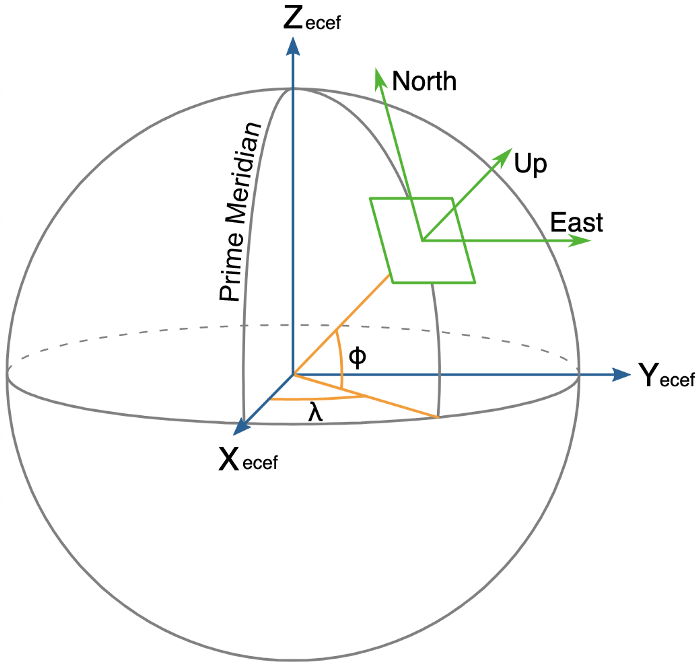### ECEF

ECEF, also known as ECR, is a geographic and Cartesian coordinate system and is sometimes known as a "conventional terrestrial" system. It represents positions as X, Y, and Z coordinates. The point is defined as the center of mass of Earth, hence the term geocentric coordinates. Read more on Wikipedia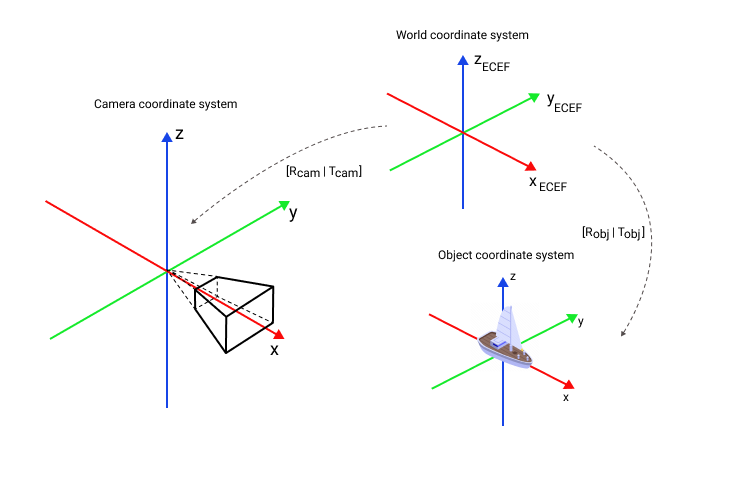### ENU

Local tangent plane coordinates (LTP) are a geographic coordinate system based on the tangent plane defined by the local vertical direction and the Earth's axis of rotation. It consists of three coordinates: one represents the position along the northern axis, one along the local eastern axis, and one represents the vertical position. The local ENU coordinates are formed from a plane tangent to the Earth's surface fixed to a specific location and hence it is sometimes known as a "Local Tangent" or "local geodetic" plane. By convention the east axis is labeled x, the north y and the up z. Read more on Wikipedia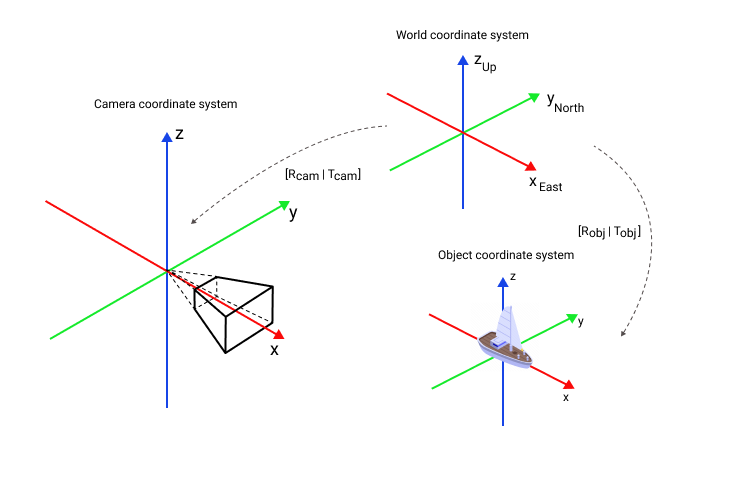# GeoPose

## Description

GeoPose is a geographically-anchored pose with 6 degrees of freedom. Position is presented as WGS-84 Geodetic point, rotation is presented as quaternion in ENU coordinate system. Example:

``````{
"position": {
"lat": 59.93930066333559,
"lon": 30.216465340943543,
"h": 6.6434114027277181
}
"quaternion": {
"w": -0.3363841028150708,
"x": 0.6584681350287606,
"y": -0.4366714830997145,
"z": -0.5124289866630708
}
}``````

## How to Convert to Cartesian Coordinate System

### Position

1. Select a reference geodetic point (lat_ref, lon_ref, h_ref), which will be the origin of your local coordinate system. For example, this could be the first position of a camera.
1. Convert geodetic position of GeoPose to position in ECEF coordinate system:
``````import math

a = 6378137
b = 6356752.3142
f = (a - b) / a
e_sq = f * (2 - f)

# Converts WGS-84 Geodetic point (lat, lon, h) to the
# Earth-Centered Earth-Fixed (ECEF) coordinates (x, y, z).
def geodetic_to_ecef(lat, lon, h):
s = math.sin(lamb)
N = a / math.sqrt(1 - e_sq * s * s)

sin_lambda = math.sin(lamb)
cos_lambda = math.cos(lamb)
sin_phi = math.sin(phi)
cos_phi = math.cos(phi)

x = (h + N) * cos_lambda * cos_phi
y = (h + N) * cos_lambda * sin_phi
z = (h + (1 - e_sq) * N) * sin_lambda

return x, y, z``````
1. Convert ECEF position to ENU position:
``````# Converts the Earth-Centered Earth-Fixed (ECEF) coordinates (x, y, z) to
# East-North-Up coordinates in a Local Tangent Plane that is centered at the
# (WGS-84) Geodetic point (lat_ref, lon_ref, h_ref).
def ecef_to_enu(x, y, z, lat_ref, lon_ref, h_ref):
s = math.sin(lamb)
N = a / math.sqrt(1 - e_sq * s * s)

sin_lambda = math.sin(lamb)
cos_lambda = math.cos(lamb)
sin_phi = math.sin(phi)
cos_phi = math.cos(phi)

x0 = (h_ref + N) * cos_lambda * cos_phi
y0 = (h_ref + N) * cos_lambda * sin_phi
z0 = (h_ref + (1 - e_sq) * N) * sin_lambda

xd = x - x0
yd = y - y0
zd = z - z0

xEast = -sin_phi * xd + cos_phi * yd
yNorth = -cos_phi * sin_lambda * xd - sin_lambda * sin_phi * yd + cos_lambda * zd
zUp = cos_lambda * cos_phi * xd + cos_lambda * sin_phi * yd + sin_lambda * zd

return xEast, yNorth, zUp``````

### Orientation

Use quaternion as is.

### Example

``````def geodetic_to_enu(lat, lon, h, lat_ref, lon_ref, h_ref):
x, y, z = geodetic_to_ecef(lat, lon, h)

return ecef_to_enu(x, y, z, lat_ref, lon_ref, h_ref)

geopose = {"position": {
"lat": 59.93930063661516,
"lon": 30.21646537256484,
"h": 6.6359911204808377
}
"quaternion": {
"w": 0.24078175147153705,
"x": 0.23898354967230406,
"y": -0.6720152706953141,
"z": -0.6582601971079732
}}

lat_ref = 59.93930066333559
lon_ref = 30.216465340943543
h_ref = 0.434114027277181

lat = geopose['position']['lat']
lon = geopose['position']['lon']
h = geopose['position']['h']

position_ref = geodetic_to_enu(lat_ref, lon_ref, h_ref, lat_ref, lon_ref, h_ref)
print(f"Reference ENU position: {position_ref}")

position = geodetic_to_enu(lat, lon, h, lat_ref, lon_ref, h_ref)
quaternion = [geopose['quaternion']['w'], geopose['quaternion']['x'],
geopose['quaternion']['y'], geopose['quaternion']['z']]

print(f'Object ENU position: {position}\nObject ENU orientation: {quaternion}')``````

Output:

``````Reference ENU position: (0.0, 0.0, 0.0)
Object ENU position: (0.0017677017435744347, -0.0029769590309327576, 6.201877094031028)
Object ENU orientation: [0.24078175147153705, 0.23898354967230406, -0.6720152706953141, -0.6582601971079732]``````

For detailed information about coordinate systems, see Geographic coordinate system.

## How to Convert to WebXR Coordinate System

1. Convert from Geopose to ENU coordinate system as shown above
2. Convert from ENU, which is a right-handed, X forward, Y to the left, Z up coordinate system, to WebXR, which is also right-handed but X to the right, Y up, Z backwards coordinate system.

Check WebXR OSCP client

### Camera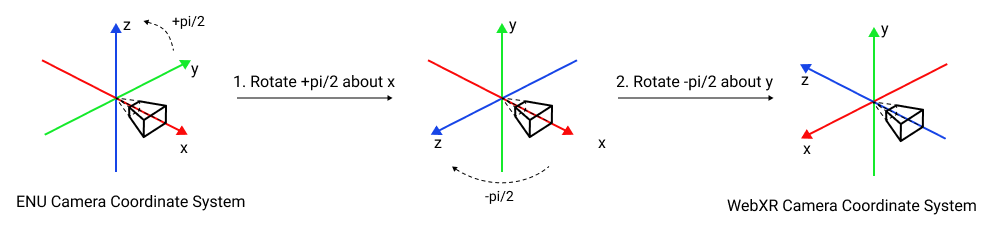### Object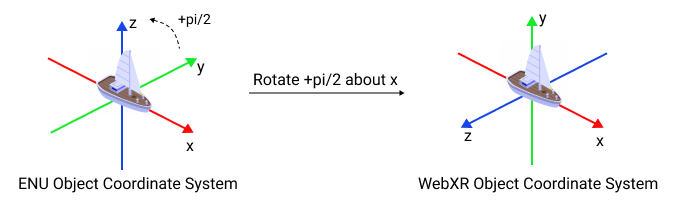## How to Convert to Unity Coordinate System

1. Convert from Geopose to ENU coordinate system as shown above
2. Convert from ENU, which is a right-handed, X forward, Y to the left, Z up coordinate system, to Unity, which is a left-handed, X to the right, Y up, Z forward coordinate system.

### Camera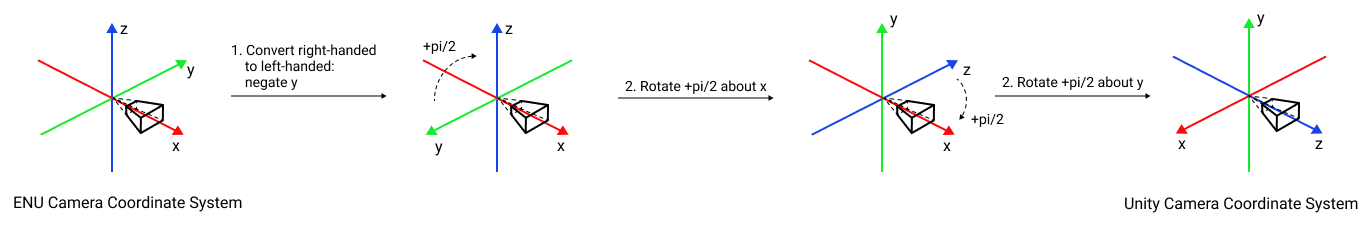### Object# OSCP API

Augmented City implementation of OSCP API

## Get camera geopose

Get camera geopose. See GeoPose

##### Request Body schema: application/json
 id required string (Id) timestamp required integer (Timestamp) The number of milliseconds since the Unix Epoch. type required string Ex. geopose. Unused property required Array of objects (Sensor) required Array of objects (SensorReading) Array of objects (GeoPoseResp) Previous geoposes. Unused property

### Request samples

Content type
application/json
`{"id": "string","timestamp": 0,"type": "string","sensors": [{"id": "string","type": "camera"}],"sensorReadings": [{"timestamp": 0,"sensorId": "string","reading": {"sequenceNumber": 0,"imageFormat": "JPG","size": [0,0],"imageBytes": "string","imageOrientation": {"mirrored": false,"rotation": 0}}}],"priorPoses": [{"id": "string","timestamp": 0,`